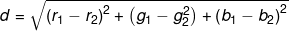# Searching for Prominent Colors in an image from a given Palette in Go — Part I

This two-part(I, II) post explores the concept of using a kd-tree data structure for identifying the prominent colors in an image from a given color palette using go.

The source code for this post is available at: https://github.com/philoj/tree-palette

## Here’s what we are trying to do:

Say we have an image of an object and we are trying to identify its color. Instead of guessing some random color, we are trying to limit our guesses to a selected list of colors. An example scenario would be analyzing the photos of products from a well-known product catalog with standard-issue colors.

## Alright, what do we know already?

Let’s say these are the choices of colors we have, let’s call it the Palette:

For each pixel in the image, we are going to choose the most similar color from the above palette. So basically, we need to convert the entire image so that all colors in the image would be from the palette.

First, we need to define what color similarity is.

The obvious approach would be to consider the fact that each color is comprised of red, blue, and green components, like a three-dimensional point. If we plot them in a graph with red, green, and blue values as the three axes, we can see that the similar ones remain close to each other:

We have our definition for similarity now: the most similar ones would then have the least distance between them!

All we need to do now is calculate the distance between different colors. In a 3d cartesian coordinate system like the one in the picture, the equation for the distance between two colors would be:where (r₁,g₁,b₁) is the first color and (r₂,g₂,b₂) is the second.

We can find the distance between each pixel’s color against each of the palette colors and find out the most similar palette color.

Now let’s write code, right?

## Wrong!

Before diving into code, let’s look at our solution once again. Say for an image of size 1920x768, and a palette size of 10. We need to compute the above equation 1920*768*10 = 1,47,45,600 times just to find out the most prominent color! Isn’t important that we take a moment to explore ways to optimize it?

Let’s see:

The problem at hand is similar to what is called a nearest neighbour search in mathematics.

What we have already discussed above is known as a linear search. There are a lot of other ways of solving this problem in the math world!

In the next part, we will explore a comparatively simpler and efficient method known as the kd-tree.. >>

--

--